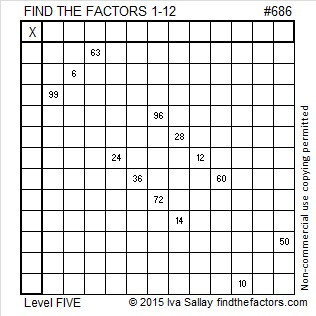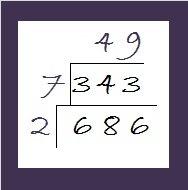# 686 and Level 5

686 is divisible by 2, not by 3, and not by 5. Is it divisible by 7? Let’s try a divisibility rule for 7’s: 68 – 2(6) = 56, a multiple of 7. Yes, 686 is divisible by 7.

686 is a palindrome in two bases:

• 686 in base 10; note that 6(100) + 8(10) + 6(1) = 681
• 222 in base 18; note that 2(324) + 2(18) + 2(1) = 681Print the puzzles or type the solution on this excel file: 12 Factors 2015-11-16

—————————————————————————————————

• 686 is a composite number.
• Prime factorization: 686 = 2 x 7 x 7 x 7, which can be written 686 = 2 x (7^3)
• The exponents in the prime factorization are 1 and 3. Adding one to each and multiplying we get (1 + 1)(3 + 1) = 2 x 4 = 8. Therefore 686 has exactly 8 factors.
• Factors of 686: 1, 2, 7, 14, 49, 98, 343, 686
• Factor pairs: 686 = 1 x 686, 2 x 343, 7 x 98, or 14 x 49
• Taking the factor pair with the largest square number factor, we get √686 = (√49)(√14) = 7√14 ≈ 26.1916017.Here is a little cake to simplify √686:Just take the square root of everything on the outside of the cake and multiply them together to get √686 = (√2)(√7)(√49) = 7√14

—————————————————————————————————